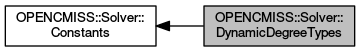OpenCMISS-Iron FORTRAN API Documentation
OPENCMISS::Solver::DynamicDegreeTypes

The time interpolation polynomial degree types for a dynamic solver. More...

Collaboration diagram for OPENCMISS::Solver::DynamicDegreeTypes:## Variables

integer(intg), parameter, public opencmiss_iron::cmfe_solver_dynamic_first_degree = SOLVER_DYNAMIC_FIRST_DEGREE
Dynamic solver uses a first degree polynomial for time interpolation. More...

integer(intg), parameter, public opencmiss_iron::cmfe_solver_dynamic_second_degree = SOLVER_DYNAMIC_SECOND_DEGREE
Dynamic solver uses a second degree polynomial for time interpolation. More...

integer(intg), parameter, public opencmiss_iron::cmfe_solver_dynamic_third_degree = SOLVER_DYNAMIC_THIRD_DEGREE
Dynamic solver uses a third degree polynomial for time interpolation. More...

## Detailed Description

The time interpolation polynomial degree types for a dynamic solver.

See also
OPENCMISS::Solver::Constants,OPENCMISS

## Variable Documentation

 integer(intg), parameter, public opencmiss_iron::cmfe_solver_dynamic_first_degree = SOLVER_DYNAMIC_FIRST_DEGREE

Dynamic solver uses a first degree polynomial for time interpolation.

See also
OPENCMISS::Solver::DynamicDegreeTypes,OPENCMISS

Definition at line 6172 of file opencmiss_iron.f90.

 integer(intg), parameter, public opencmiss_iron::cmfe_solver_dynamic_second_degree = SOLVER_DYNAMIC_SECOND_DEGREE

Dynamic solver uses a second degree polynomial for time interpolation.

See also
OPENCMISS::Solver::DynamicDegreeTypes,OPENCMISS

Definition at line 6173 of file opencmiss_iron.f90.

 integer(intg), parameter, public opencmiss_iron::cmfe_solver_dynamic_third_degree = SOLVER_DYNAMIC_THIRD_DEGREE

Dynamic solver uses a third degree polynomial for time interpolation.

See also
OPENCMISS::Solver::DynamicDegreeTypes,OPENCMISS

Definition at line 6174 of file opencmiss_iron.f90.